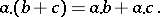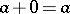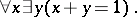# Semi-ring

(diff) ← Older revision | Latest revision (diff) | Newer revision → (diff)

A non-empty set with two associative binary operationsand, satisfying the distributive lawsandIn most cases one also assumes that the addition is commutative and that there exists a zerosuch thatfor every. The most important examples of semi-rings are rings and distributive lattices (cf. Ring; Distributive lattice). If there is a multiplicative identity 1, the two classes are combined by the conditionThe non-negative integers with the usual operations provide an example of a semi-ring that does not satisfy this condition.

How to Cite This Entry:
Semi-ring. Encyclopedia of Mathematics. URL: http://encyclopediaofmath.org/index.php?title=Semi-ring&oldid=16685
This article was adapted from an original article by L.A. Skornyakov (originator), which appeared in Encyclopedia of Mathematics - ISBN 1402006098. See original article